# How to Calculate and Solve for Relative Freezing Time | Foundry Technology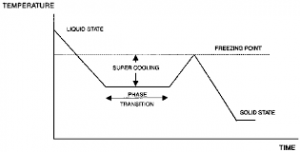The image above represents relative freezing time.

To compute for relative freezing time, four essential parameters are needed and these parameters are Constant (L), Constant (C), Volume of Riser/Volume of Casting (y) and Relative Contraction of Freezing (B).

The formula for calculating relative freezing time:

x = L / y – B + C

Where:

x = Relative Freezing Time
y = Volume of Riser / Volume of Casting
B = Relative Contraction on Freezing
C = Constant
L = Constant

Let’s solve an example;
Find the relative freezing time when the volume of riser/volume of casting is 26, the relative contraction on freezing is 21, the constant is 8 and the constant is 11.

This implies that;

y = Volume of Riser / Volume of Casting = 26
B = Relative Contraction on Freezing = 21
C = Constant = 11
L = Constant = 8

x = L / y – B + C
x = 8 / 26 – 21 + 11
x = 8 / 5 + 11
x = 1.6 + 11
x = 12.6

Therefore, the relative freezing time is 12.6 s.

Calculating the Constant (L) when the Relative Freezing Time, Constant (C), the Volume of riser/Volume of Casting and the Relative Contraction on Freezing is Given.

L = x (y – B) – C

Where;

L = Constant
x = Relative Freezing Time
y = Volume of riser / Volume of Casting
B = Relative Contraction on Freezing
C = Constant

Let’s solve an example;
Find the Constant when the relative freezing time is 24, the volume of riser / volume of casting is 14, the relative contraction on freezing is 8 and the constant is 10.

This implies that;

x = Relative Freezing Time = 24
y = Volume of riser / Volume of Casting = 14
B = Relative Contraction on Freezing = 8
C = Constant = 10

L = x (y -B) – C
L = 24 (14 – 8) – 10
L = 24 (6) – 10
L = 144 – 10
L = 134

Therefore, the constant is 134.

Calculating the Constant (C) when the Relative Freezing Time, the Constant (L), the Volume of Riser / Volume of Casting and the Relative Contraction on Freezing is Given.

C = x (y – B) – L

Where;

C = Constant
x = Relative Freezing Time
y = Volume of Riser / Volume of Casting
B = Relative Contraction on Freezing
L = Constant

Let’s solve an example;
Find the constant when the relative freezing time is 5, the volume of riser / volume of casting is 10, the relative contraction on freezing is 2 and the constant is 4.

This implies that;

x = Relative Freezing Time = 5
y = Volume of Riser / Volume of Casting = 10
B = Relative Contraction on Freezing = 2
L = Constant = 4

C = x (y – B ) – L
C = 5 (10 – 2) – 4
C = 5 (8) – 4
C = 40 – 4
C = 36

Therefore, the constant is 36.

Calculating the Volume of Riser / Volume of Casting when the Relative Freezing Time, the Constant (L), the Constant (C) and the Relative Contraction on Freezing is Given.

y = L + C / x + B

Where;

y = Volume of Riser / Volume of Casting
L = Constant
C = Constant
x = Relative Freezing Time
B = Relative Contraction on Freezing

Let’s solve an example;
Given that the constant is 12, the relative freezing time is 6, the constant is 24 and the relative contraction on freezing is 3. Find the Volume of riser / Volume of casting?

This implies that;

L = Constant = 12
C = Constant = 24
x = Relative Freezing Time = 6
B = Relative Contraction on Freezing = 3

y = L + C / x + B
y = 12 + 24 / 6 + 3
y = 36 / 6 + 3
y = 6 + 3
y = 9

Therefore, the volume of riser / volume of casting is 9.

Calculating the Relative Contraction on Freezing when the Relative Freezing Time, the Constant (L), the Constant (C) and the Volume of Riser / Volume of Casting is Given.

B = y – L + C / x

Where;

B = Relative Contraction on Freezing
y = Volume of Riser / Volume of Casting
L = Constant
C = Constant
x = Relative Freezing Time

Let’s solve an example;
Find the relative contraction on freezing when the volume of riser / volume of casting is 32, the constant is 20, the constant is 12 and the relative freezing time is 8.

This implies that;

y = Volume of Riser / Volume of Casting = 32
L = Constant = 20
C = Constant = 12
x = Relative Freezing Time = 8

B = y – L + C / x
B = 32 – 20 + 12 / 8
B = 32 – 32 / 8
B = 32 – 4
B = 28

Therefore, the relative contraction on freezing is 28.

Nickzom Calculator – The Calculator Encyclopedia is capable of calculating the relative freezing time.

To get the answer and workings of the relative freezing time using the Nickzom Calculator – The Calculator Encyclopedia. First, you need to obtain the app.

You can get this app via any of these means:

To get access to the professional version via web, you need to register and subscribe for NGN 1,500 per annum to have utter access to all functionalities.
You can also try the demo version via https://www.nickzom.org/calculator

Apple (Paid) – https://itunes.apple.com/us/app/nickzom-calculator/id1331162702?mt=8
Once, you have obtained the calculator encyclopedia app, proceed to the Calculator Map, then click on Materials & Metallurgical under Engineering.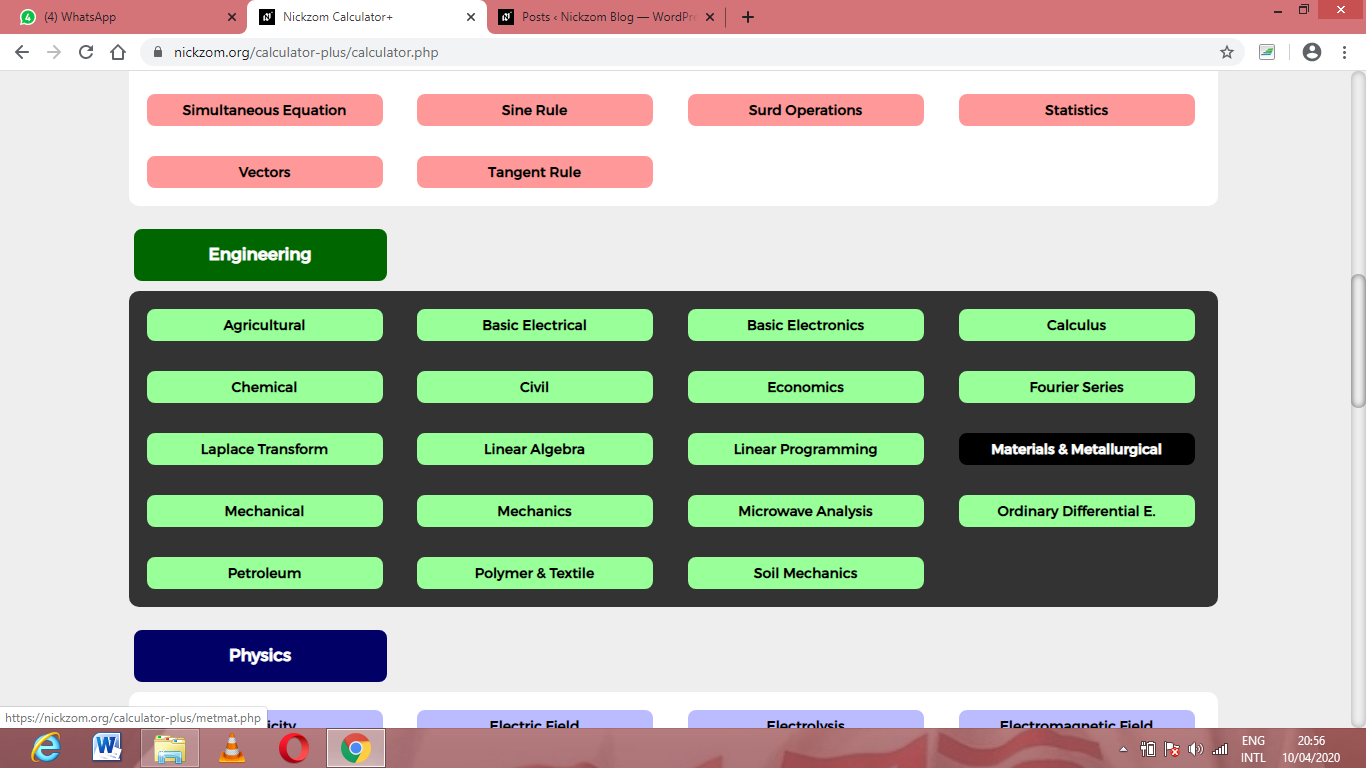Now, Click on Foundry Technology under Material & MetallurgicalNow, Click on Relative Freezing Time under Foundry TechnologyThe screenshot below displays the page or activity to enter your values, to get the answer for the relative freezing time according to the respective parameters which are the Constant (L), Constant (C), Volume of Riser/Volume of Casting (y) and Relative Contraction of Freezing (B).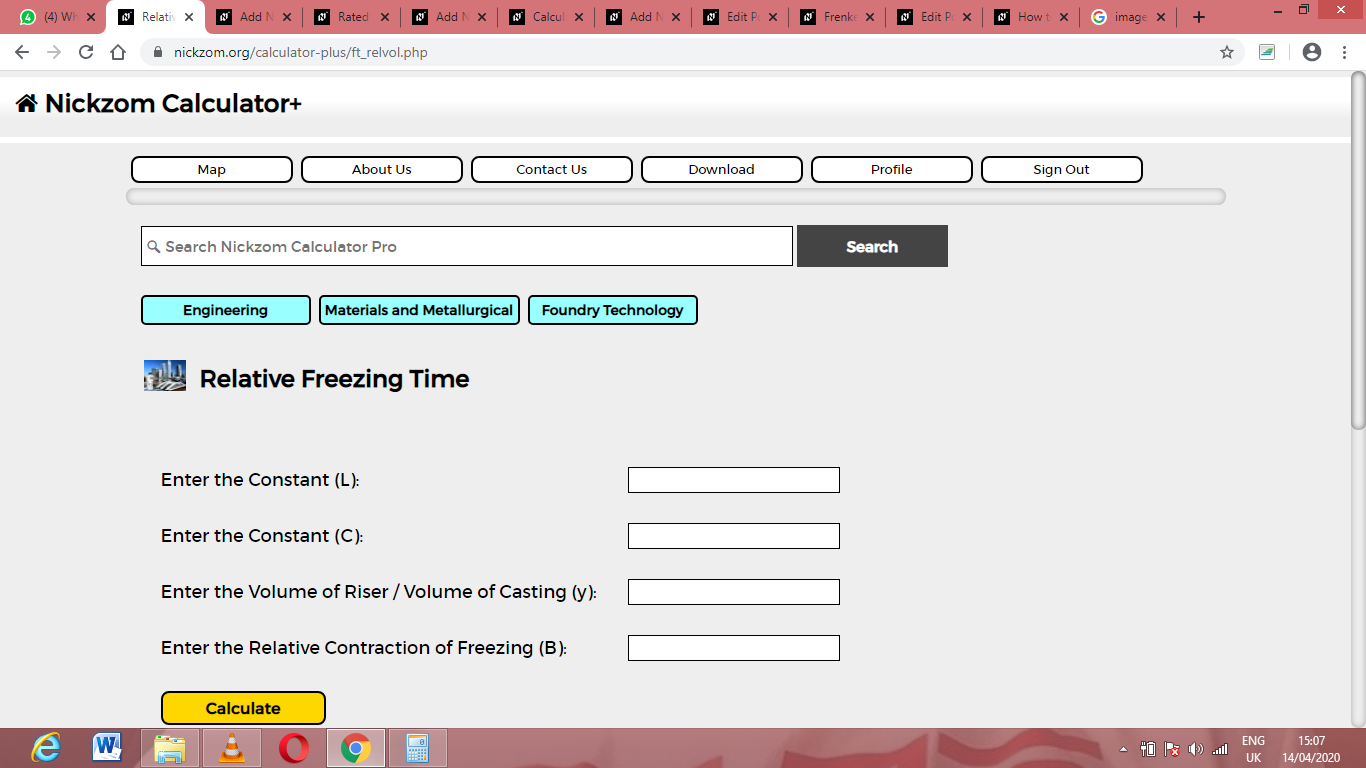Now, enter the values appropriately and accordingly for the parameters as required by the Constant (L) is 8, Constant (C) is 11, Volume of Riser/Volume of Casting (y) is 26 and Relative Contraction of Freezing (B) is 21.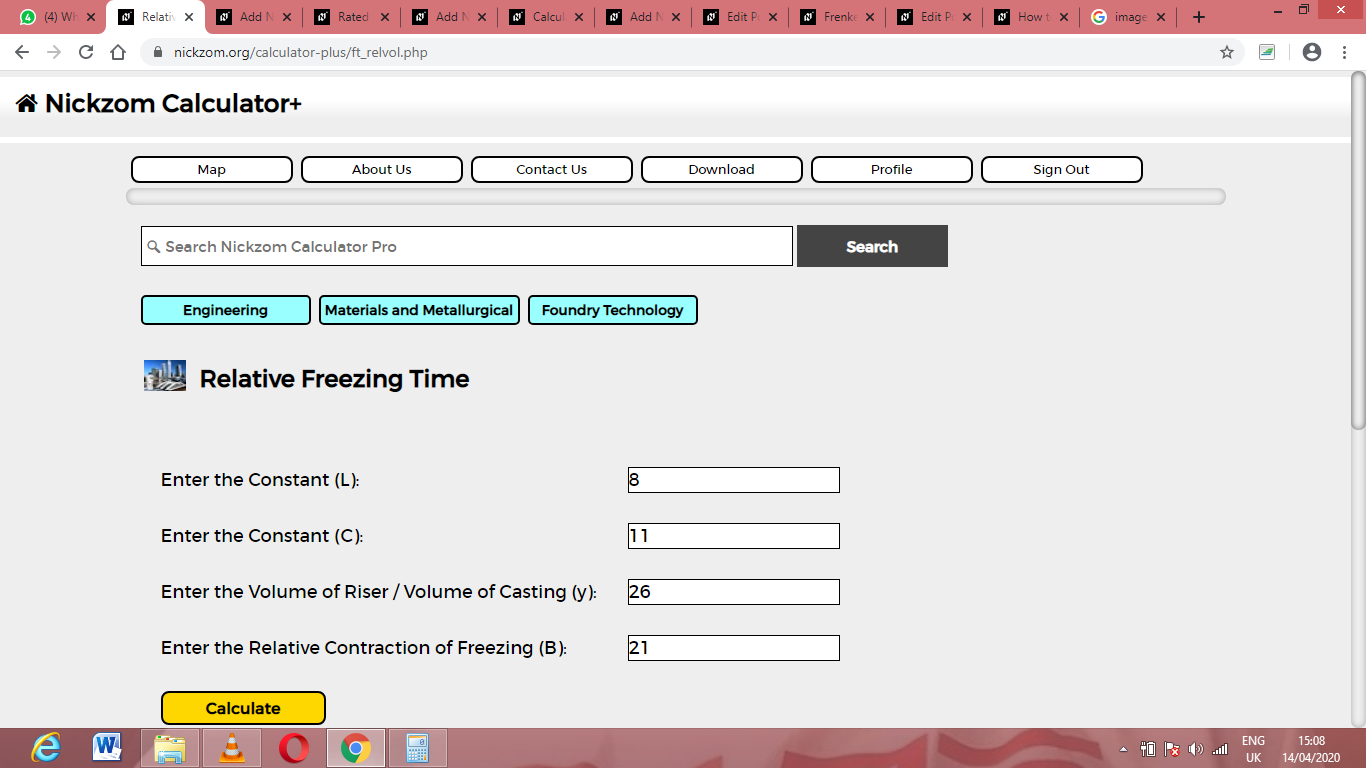Finally, Click on Calculate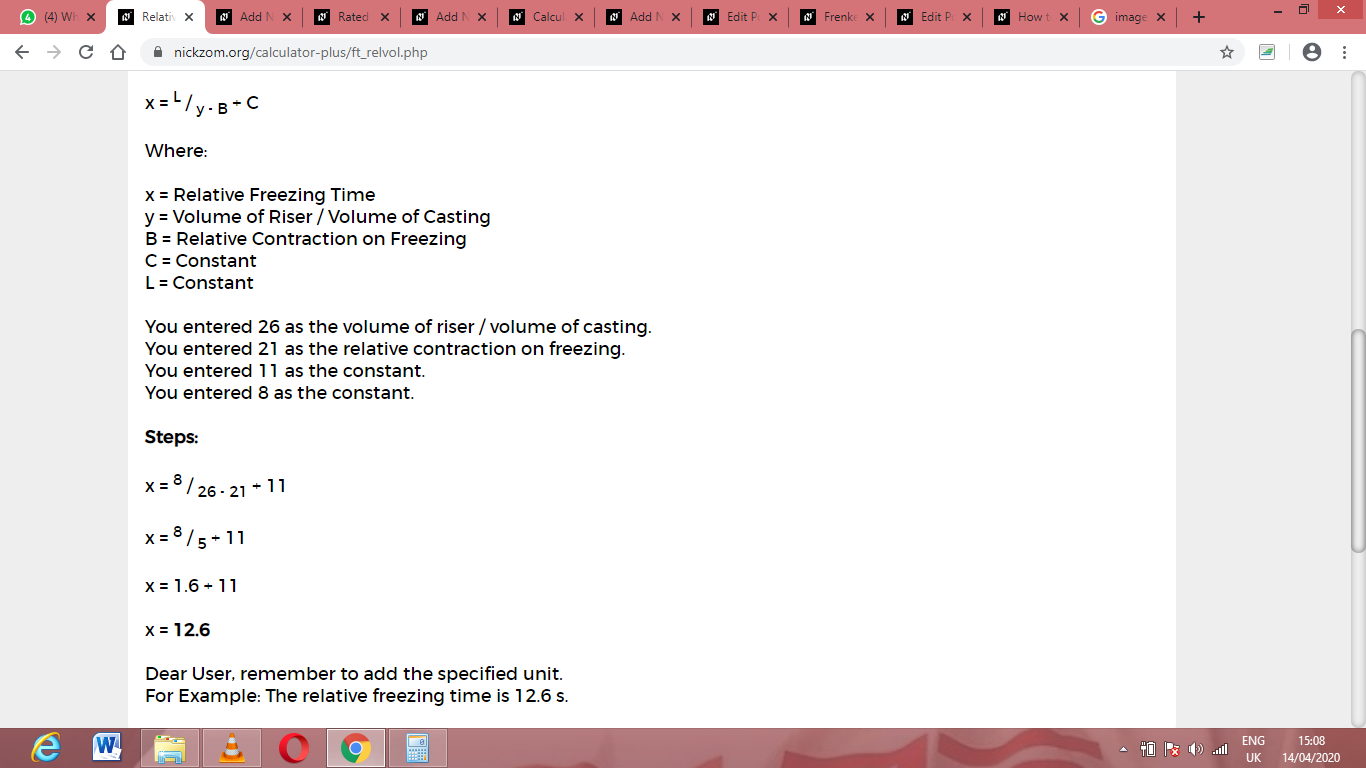As you can see from the screenshot above, Nickzom Calculator– The Calculator Encyclopedia solves for the relative freezing time and presents the formula, workings and steps too.